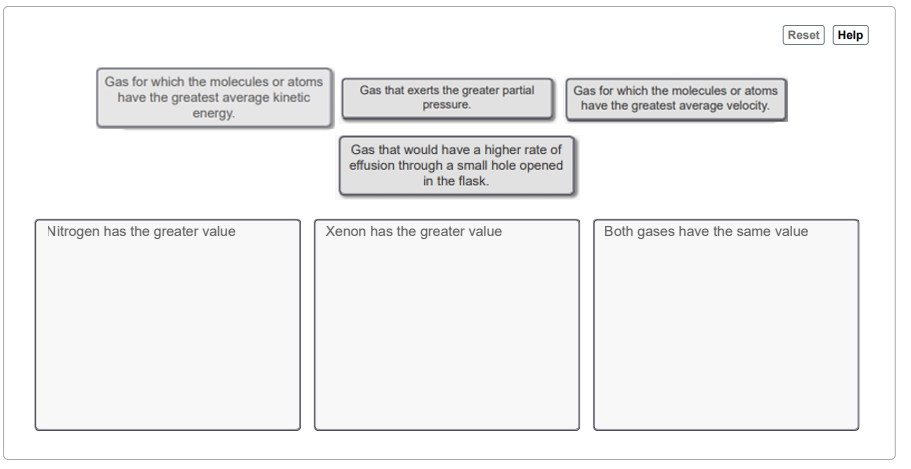# Problem: You may want to reference (Pages 224 - 230) Sections 5.8 while completing this problem.A flask at room temperature contains exactly equal amounts (in moles) of nitrogen and xenon. Sort the conditions based on the gas described.  Drag the appropriate items to their respective bins.

###### FREE Expert Solution

Recall that Graham's Law of Effusion allows us to compare the rate of effusion of two gases. Graham's Law states that the rate of effusion of a gas is inversely proportional to its molar mass.

$\mathbf{rate}\mathbf{=}\frac{\mathbf{1}}{\sqrt{{\mathbf{MM}}_{\mathbf{gas}}}}$

The partial pressure and the average kinetic energy of a gas are not related to the amount of the gas particles. The rate of effusion and the average velocity of gas particles decreases as the molar mass of the particles increases.

Molar mass of N2 : 28 g/mol → lighter → faster rate of effusion

Molar mass of Xe: 131.29 g/mol → heavier → slower rate of effusion###### Problem Details

You may want to reference (Pages 224 - 230) Sections 5.8 while completing this problem.

A flask at room temperature contains exactly equal amounts (in moles) of nitrogen and xenon. Sort the conditions based on the gas described.  Drag the appropriate items to their respective bins.